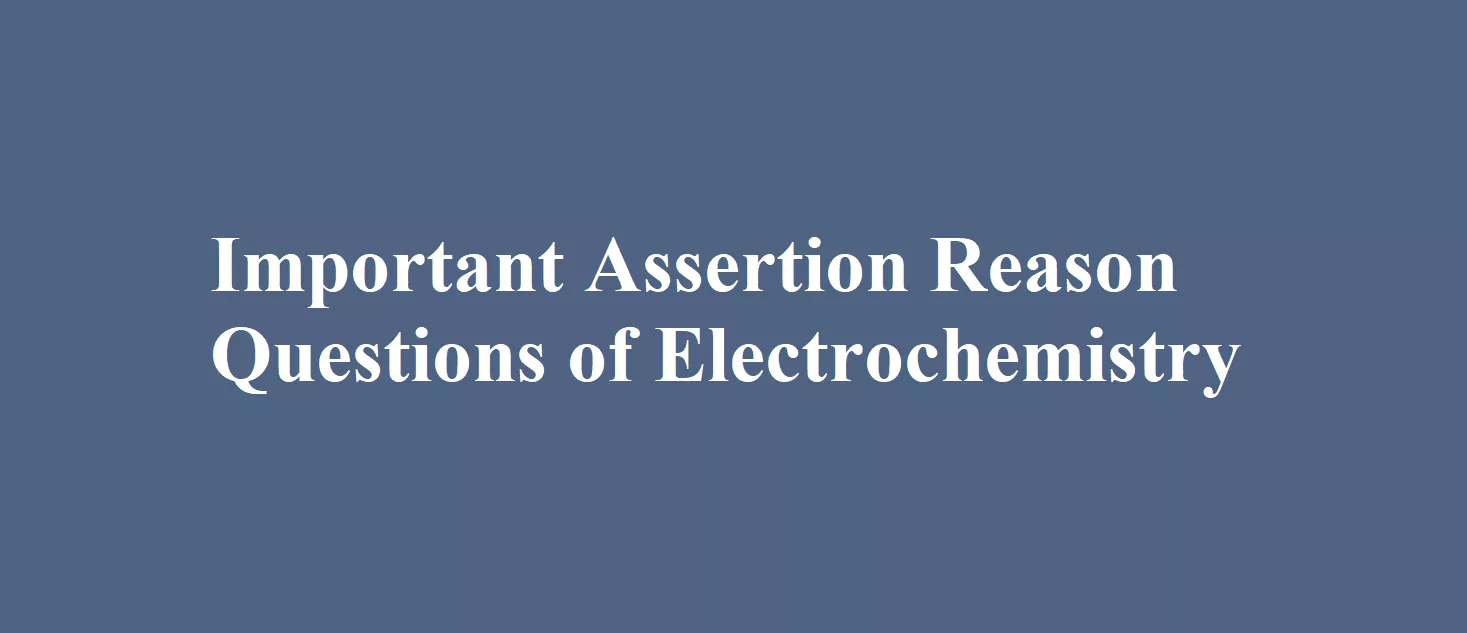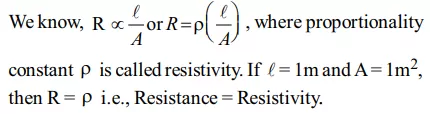# Important Assertion Reason Questions of ElectrochemistryImportant Assertion Reason Questions of Electrochemistry

Que 1. Assertion: In an electrochemical cell anode and cathode are respectively negative and positive electrodes.
Reason: At anode oxidation takes place and at cathode reduction takes place.
Ans 1. (a) Both assertion and reason are true and the reason is the correct explanation of assertion.
Explanation: Anode (oxidation) for eq Zn → Zn2+ + e
Excess of electrons and hence negatively charged while the cathode is positively charged.

Que 2. Assertion: Galvanised iron does not rust.
Reason: Zn has more (–) ve electrode potential than Fe.
Ans 2. (a)

Que 3. Assertion: Conductivity decreases with dilution.
Reason: The number of ions per unit volume decreases on dilution.
Ans 3. (a)

Important Assertion Reason Questions of Electrochemistry

Que 4. Assertion: Ni/Ni2+(1.0M)||Au3+(1.0M)|Au , for this cell emf is 1.75 V if Eo Au3+|Au = 1.50, EoNi/Ni2+ = – 0.25 .
Reason: Emf of the cell = Eo cathode – Eoanode.
Ans 4. (a) Both assertion and reason are true and the reason is the correct explanation of assertion.
Explanation: Eo Au3+|Au – EoNi/Ni2+ = 1.50 – (-0.25) = 1.75 V.

Que 5. Assertion: The resistivity for a substance is its resistance when it is one meter long and its area of cross-section is one square meter.
Reason: The SI units of resistivity is ohm meter ( m)
Ans 5. (b)
Explanation:Que 6. Assertion: Salts like KCl, KNO3 i.e., inert electrolytes are used in salt bridge.
Reason: An inert electrolyte can easily be filled in the Utube.
Ans 6. (c) Assertion is true but the reason is false.
Explanation: Ions of inert electrolytes are not involved in any electrochemical change until they react chemically with the electrolytes in the two half-cells.

Important Assertion Reason Questions of Electrochemistry

Que 7. Assertion: On increasing dilution, the specific conductance keeps on increasing.
Reason: On increasing dilution, the degree of ionisation of weak electrolyte increases, and molality of ions also increases.
Ans 7. (d)
Explanation: The specific conductivity decreases while equivalent and molar conductivities increase with dilution.

Que 8.  Assertion: Emf and potential difference are the same for cells.
Reason: Both give the difference in electrode potential under any condition.
Ans 8. (d) Both assertion and reason are false.
Explanation: Potential difference is the difference between the electrode potential of the two electrodes of the cell when the cell is under operation while emf is the potential difference generated by a cell when there is zero electron flow.

Que 9. Assertion: Galvanised iron does not rust.
Reason: Zinc has a more negative electrode potential than iron.
Ans 9.  (a)
Explanation: Zinc metal which has a more negative electrode potential than iron will provide electrons in preference of the iron, and therefore corrodes first. Only when all the zinc has been oxidised, the iron start to rust.

Que 10. Assertion: Current stops flowing when Ecell = 0.
Reason: Equilibrium of the cell reaction is attained.
Ans 10. (a)

Important Assertion Reason Questions of Electrochemistry